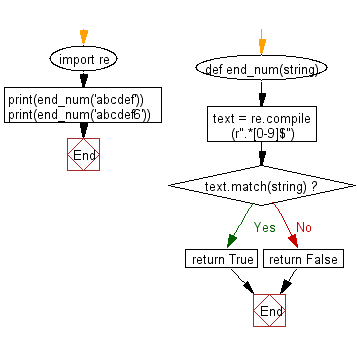﻿ Python: Check for a number at the end of a string - w3resource# Python: Check for a number at the end of a string

## Python Regular Expression: Exercise-17 with Solution

Write a Python program to check for a number at the end of a string.

Sample Solution:-

Python Code:

``````import re
def end_num(string):
text = re.compile(r".*[0-9]\$")
if text.match(string):
return True
else:
return False

print(end_num('abcdef'))
print(end_num('abcdef6'))
```
```

Sample Output:

```False
True
```

Pictorial Presentation:Flowchart:## Visualize Python code execution:

The following tool visualize what the computer is doing step-by-step as it executes the said program:

Python Code Editor:

Have another way to solve this solution? Contribute your code (and comments) through Disqus.

What is the difficulty level of this exercise?

Test your Programming skills with w3resource's quiz.

﻿

## Python: Tips of the Day

Combining two iterable of tuples or pivot nested iterables:

```# Combining two iterables
>>> a = [1, 2, 3]
>>> b = ['a', 'b', 'c']
>>> z = zip(a, b)
>>> z
[(1, 'a'), (2, 'b'), (3, 'c')]

# Pivoting list of tuples
>>> zip(*z)
[(1, 2, 3), ('a', 'b', 'c')]
```